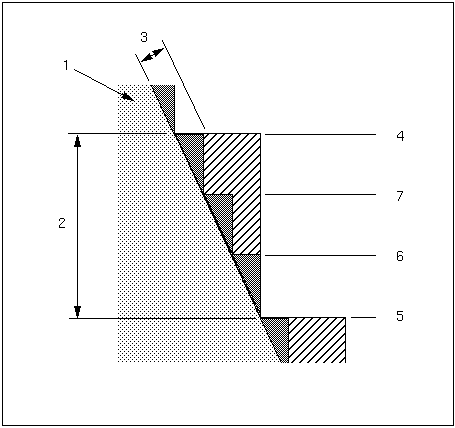Manufacturing > Manufacturing > Milling > Roughing and Reroughing > Generating Extra Slices for Scallop Control
Generating Extra Slices for Scallop Control
You can use the ROUGH_SCALLOP_CONTROL and ROUGH_SCALLOP parameters to control the scallop height left on surfaces after a Roughing NC sequence. The ROUGH_SCALLOP parameter specifies the maximum allowed scallop height measured in a direction normal to the surface.
The system first calculates the uniform step depth and generates the machining slices according to this step depth value. If the resulting scallop height exceeds the ROUGH_SCALLOP parameter value, the system adds extra slices where required, as shown in the following diagram. These extra slices can either be interspersed with the uniform machining slices, or all be performed at the end, after the uniform machining is finished, depending on the ROUGH_SCALLOP_CONTROL parameter value.
The following diagram illustrates the order of generating slices if ROUGH_SCALLOP_CONTROL is set to DURING_BOTTOM_UP.1. Reference part
2. Uniform step depth <= MAX_STEP_DEPTH
3. ROUGH_SCALLOP, measured in the direction normal to the part surface
The tool machines the uniform slices, 4 and 5. Slice 5 results in a scallop exceeding the ROUGH_SCALLOP value, the system generates the extra slices, 6 and 7, to decrease the scallop height. These slices are machined from the bottom up, that is, slice 6 is machined first, and then slice 7, to optimize the tool load. Then the tool moves to the next uniform slice, step depth below the slice 5, and so on.
If ROUGH_SCALLOP_CONTROL is set to DURING, the system generates the same extra slices, but the order of machining is different. The tool machines the uniform slices, 4 and 5, and then the extra slices from the top down, first slice 7 and then slice 6. Then the tool moves to the next uniform slice, step depth below the slice 5, and so on.
If ROUGH_SCALLOP_CONTROL is set to AFTER, the tool machines all the uniform slices first, and then machines the extra slices to reduce the scallop height, where needed.